neuronTypes slides

# Neuron types¶

## Output units¶

• The activation function of the neurons should correspond to the error function.

• The important point is that we want to prevent saturation which results in very slow learning.

The output unit type is dependent on the task:

• for classification:
• sigmoid neuron (two classes)
• softmax (multiple exclusive classes)
• for regression:
• linear (identity)

### Example: Binary Classification¶

For a classifiation problem with two classes the output $o$ of a sigmoid unit predicts the probability of class 1:

$$o(\vec x) = p(y=1 \mid \vec x; \theta)$$

The appropriate loss (function) for example $k$ is cross entropy:

$$J^{(k)} (\vec \theta) = - t^{(k)} \log o(\vec x^{(k)}) - (1-t^{(k)}) \log (1-o(\vec x^{(k)}))$$

The exponentiation in the logistic function and the logarithm of the loss function cancels out. The derivation of $J^{(k)} (\vec \theta)$ for adapting the output weights $\theta^{(l)}$ will become:

$$\frac{J^{(k)}(\vec \theta)}{\partial \vec \theta^{(l)}} \propto (o(\vec x^{(k)}) - t^{(k)})$$

"natural pairing of error function and output unit activation function, which gives rise to this simple form for the derivative." [Bis95, p. 232]

## Hidden Units¶

The "classical" neuron types for hidden units is the tanh (see e.g. [Le98]).

Recently other units are used, e.g.

• Rectified Linear Units (relu) [Glo11]
• Maxout [God13]

#### Sigmoid units¶

In :
# the sigmoid function:
def sigmoid(z):
return 1./(1 + np.exp(-z))

plot_func(sigmoid, "Sigmoid", (-.1, 1.1))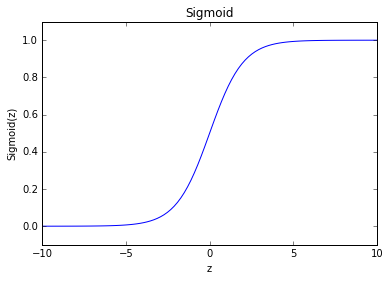For small or large input values the derivation is nearly zero (saturation). Therefore learning with first order methods is in this range nearly impossible.

#### Tanh¶

In :
plot_func(np.tanh, "Tangens Hyperbolicus", (-1.1, 1.1))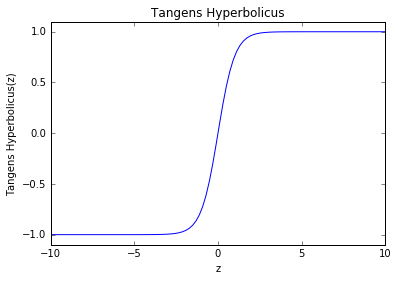#### Modification of tanh which don't saturate¶

proposed by Yann LeCun [Le89]

In :
def tanh_mod(z, a = 0.02):
return 1.7159 * np.tanh(2./3 * z) + a * z

plot_func(tanh_mod, "mod. tanh", (-2.1, 2.1))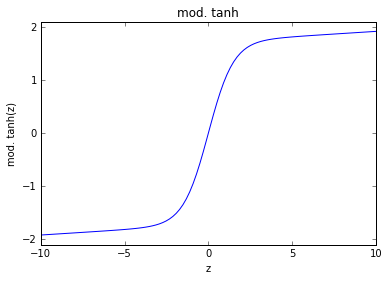#### Rectified Linear Units¶

A neuron type which has no saturation for positive input is the rectified linear unit [Glo11].

In :
def linear_rectified(z):
return np.maximum(0, z)

plot_func(linear_rectified, "ReLu", (-.1, 10.))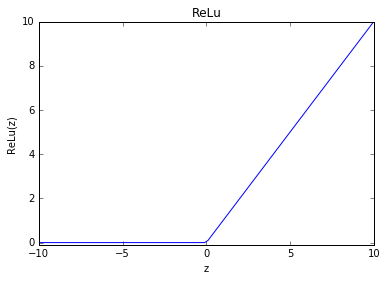### Leaky Rectified Linear Units¶

In :
def leaky_linear_rectified(z, a = .01):
return np.maximum(0, z) + a * np.minimum(0, z)

plot_func(linear_rectified, "Leaky ReLu", (-.2, 10.))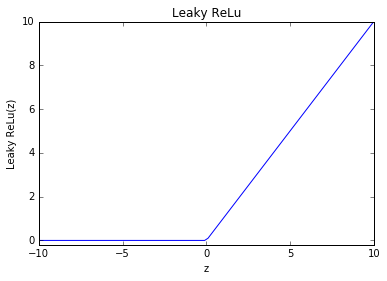### Softplus¶

Softplus is a smooth variant of the linear rectified unit:

$$a(z) = \log(1. + e^z)$$
In :
def softplus(z):
return np.log(1.+np.exp(z))

plot_func(softplus, "Softplus", (-.2, 10))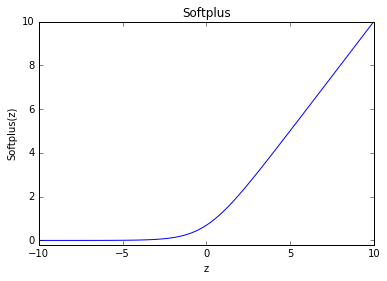### Literature¶

• [Bis95] Bishop, Christopher M. Neural networks for pattern recognition. Oxford university press, 1995.
• [Glo11] X. Glorot, "Deep Sparse Rectifier Neural Networks (2011)
• [God13] Ian J. Goodfellow , David Warde-farley , Mehdi Mirza , Aaron Courville , Yoshua Bengio: Maxout Networks, ICML, 2013
• [Le98] Y. LeCun, L. Bottou, G. Orr and K. Muller: Efficient BackProp, in Orr, G. and Muller K. (Eds), Neural Networks: Tricks of the trade, Springer, 1998
≈‚